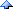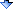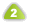# 77家的会客厅2010

PYTHON实现阶乘算法
Weather:忘了啥了,反正挺舒服

def f(n):
c = 1
for i in range(n+1):
c  *= i
return c

def f(n):
if n > 1:
return n*f(n-1)
else:
return 1

def f(n):
p = 1
if n > 1:
p = n*f(n-1)
return p

def foo(n):
return reduce(lambda x,y: x*y, range(1,n+1))

----------------------我是转载分隔线----------------------

['a.b.c.d11u.e.f.g', 'e.f88.g', 'caa3.z.brr', 'z.48.ff.ee']

node_list = ['a.b.c.d11u.e.f.g', 'e.f88.g', 'caa3.z.brr', 'z.48.ff.ee']
max_node = max(node_list, key=lambda n: n.count('.'))

node_list = ['a.b.c.d11u.e.f.g', 'e.f88.g', 'caa3.z.brr', 'z.48.ff.ee']
max_node = reduce(lambda a,b: a.count('.') >= b.count('.') and a or b, node_list)

reduce接受两个必选参数，第一个参数是一个函数，这个函数接受两个参数，返回一个值；第二个参数是一个序列（比如列表）; reduce首先把序列的前两个元素作为参数传递给参数函数，然后把参数函数的返回值和序列的第三个参数一起再传递给参数函数，依次类推，最终只剩下一个值。这么解释似乎更晕啦，哈哈，还是python自己的help说的好：For example, reduce(lambda x, y: x+y, [1, 2, 3, 4, 5]) calculates ((((1+2)+3)+4)+5)(04/08) 用VBA实现EXCEL单元格输入即保护功能
Python中的正则表达式入门(1) (04/11)[2005/04/10]做了个雨中疯子,终于有了同居对象

### [PYTHON实现阶乘算法]的回复re 于 2009-04-10 01:09:23 发表 | IP:114.93.108.*
Python不支持尾递归吧？写这种玩意需要换个思路，和一般的不一样。柠檬园主 于 2009-04-10 01:28:53 发表 | IP:119.109.24.*
PYTHON弱类型,定义的函数没有明确类型,所以在这儿没办法递归.... :(柠檬园主 于 2009-04-10 09:35:22 发表 | IP:116.3.197.*

4#   quake0day 于 2010-03-11 03:30:21 发表 | IP:219.232.107.*
1 def f(n):
2     c = 1
3    for i in range(1,n+1):
4 c *= i
5 return c

5#   any 于 2015-05-25 11:24:36 发表 | IP:221.222.242.*
python是支持尾递归的，不过要把acc累计 放入其他fun
6#   any 于 2015-05-25 11:25:05 发表 | IP:221.222.242.*
def f(n):
return k(n-1,acc)

def k(i,acc):
if i ==1:
return j
return k(i-1,acc*i)

` def f(n, acc=1):    if n == 0:        return acc    return f(n-1, acc * n)`

```def f(n, ret1=0, ret2=1):
if n < 2:
return ret1
return f(n-1, ret2, ret1+ret2)```

```def f1(n):
if n < 2:
return n
return f1(n-1) + f1(n-2)```

但是如果直接用循环写的话，即使是和尾递归比起来，也会有“飞”一般的感觉

```def f2(num):
a, b = 0, 1
for n in xrange(num):
L = a
a, b = b, a+b
return L
```